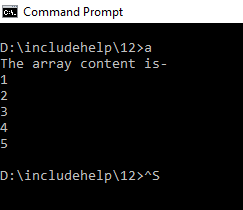# fread() function in C language with Example

Here, we are going to learn about the fread() function of library header stdio.h in C language with its syntax, example.
Submitted by Souvik Saha, on January 11, 2019

Prototype:

```    size_t fread(void *buffer, size_t length, size_t count, FILE *filename);
```

Parameters:

```    void *buffer, size_t length, size_t count, FILE *filename
```

Return type: size_t

Use of function:

The prototype of the function fread() is:

```    size_t fread(void *buffer, size_t length, size_t count, FILE *filename);
```

In the file handling, through the fread() function, we read the count number of objects of size length from the input stream filename to the array named buffer. Its returns the number of objects being read from the file. If lesser no of objects are read or EOF is encountered before then it will give an error.

```#include <stdio.h>
#include <stdlib.h>

int main()
{
FILE* f;
//initialize the arr1 with values
int arr1 = { 1, 2, 3, 4, 5 };
int arr2;
int i = 0;

//open the file for write operation
if ((f = fopen("includehelp.txt", "w")) == NULL) {
//if the file does not exist print the string
printf("Cannot open the file...");
exit(1);
}
//write the values on the file
if ((fwrite(arr1, sizeof(int), 5, f)) != 5) {
printf("File write error....\n");
}
//close the file
fclose(f);

//open the file for read operation
if ((f = fopen("includehelp.txt", "r")) == NULL) {
//if the file does not exist print the string
printf("Cannot open the file...");
exit(1);
}
//read the values from the file and store it into the array
if ((fread(arr2, sizeof(int), 5, f)) != 5) {
printf("File write error....\n");
}
fclose(f);

printf("The array content is-\n");
for (i = 0; i < 5; i++) {
printf("%d\n", arr2[i]);
}

return 0;
}
```

Output